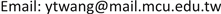﻿ 区域经济整合与资本外流 Regional Economic Integration and Capital Outflow

World Economic Research
Vol. 07  No. 03 ( 2018 ), Article ID: 26878 , 8 pages
10.12677/WER.2018.73011

Regional Economic Integration and Capital Outflow

Yuter Wang

Department of Economics, Ming Chuan University, Taipei TaiwanReceived: Aug. 26th, 2018; accepted: Sep. 13th, 2018; published: Sep. 20th, 2018ABSTRACT

Using a general equilibrium model with specific factors, the present paper studies the welfare impact on the investing country itself, its partners, the economic region as a whole and the recipient as well as the whole world as a member country invests in the integrated region. I find that an increase in the capital outflow will harm the member itself, when the product of the investing country’s share of the receiving country’s capital stock and the recipient’s capital return elasticity is greater than one as well as the member country owns some capital from partners initially. The partners’ welfare will rise in the presence of the large capital outflow to the member investing outside. The imposition of tariff or capital tax will make the nonmember country lose from the increased capital inflow. A lower common tariff will ensure the economic region and the world benefits from the capital flow.

Keywords:Regional Economic Integration, Capital Flow, General Equilibrium Analysis1. 引言

  已在典型的两国模型中分析资本外移的福利效果，在其Heckscher-Ohlin 模型设定下，要素之报酬只是商品价格的函数，并不因要素本身数量的增减而改变，他证明，当出口商品是资本密集，资本外流是不利的。然而，这些传统文献并无法具体显现出经济整合之下同盟国彼此之间休戚相关、对外一致的特性，所以一些因经济结合而产生的效果将不免漏失。国际贸易理论一般认为关税同盟(customs union)是一些国家彼此结合，对于内部贸易不加任何限制，对外则设立一致的关税结构。由于生产要素开放迁移为经济整合的必经过程，欧体并且已于1993年实施，因此在经济整合之下探讨要素移动理论应将此特性列入考虑。但为了将分析焦点集中在资本流动之上，本文不拟讨论劳动迁徙的问题。在本文中，所将考虑的经济区域是一个允许资本自由移动的关税同盟。因此，会员国的资本出口将透过区域内的资本移动与共同对外设置的关税影响盟国的福利，此时会员国福利的改变将与传统分析有所区别。另外，整个区域与世界福利所受之影响亦有探讨的必要。在传统的Heckscher-Ohlin架构下，会员国的资本外流对整合区域内的资本市场不具影响，本文中会员国间资本自由移动的特性在此将无意义2。另一方面，鉴于外人直接投资经常发生在进口竞争产业，我们因此将资本限制在被投资之非会员国的进口部门，道种设定亦不乏文献采用，如   与  。基于这些目的，会员国的资本外流将被视为外生给定，此种讨论资本移动的方式亦为       与  等所采用，优点在于可清楚地看出直接由资本移动所引起的效果，这有别于藉由提高关税保护来考虑间接诱发的内生性资本移动效果    。另外，我们将组合  的特定要素(specific factor)模型架构与  的分析方式进行讨论。本文其余部份的结构如下：第二节建立我们的三国两商品三要素一般均衡模型。第三节首先讨论投资国、其盟国与整个经济区域由此投资获利或受损的情况；其次探讨会员国的投资是否必然有利于受投资之非会员国；最后，并就世界福利所受影响进行分析。第四节整理归纳本文的重要结果。

2. 基础模型

${k}^{a}={\overline{k}}^{a}-i-{i}^{c}$ (1)

${k}^{b}={\overline{k}}^{b}+i$ (2)

${k}^{c}={\overline{k}}^{c}+i$ (3)

$R={r}_{k}^{a}\left(p,{k}^{a}\right)={r}_{k}^{b}\left(p,{k}^{b}\right)$ (4)

${e}^{a}\left(p,{u}^{a}\right)={r}^{a}\left(p,{k}^{a}\right)+\left(1-\omega \right){r}_{k}^{c}{i}^{c}+iR+t\left({e}_{p}^{a}-{r}_{p}^{a}\right)$ (5)

${e}^{b}\left(p,{u}^{b}\right)={r}^{b}\left(p,{k}^{b}\right)-iR+t\left({e}_{p}^{b}-{r}_{p}^{b}\right)$ (6)

${e}^{c}\left(p,{u}^{c}\right)={r}^{c}\left(p,{k}^{c}\right)-\left(1-\omega \right){r}_{k}^{c}{i}^{c}$ (7)

3. 结盟国资本外流之效益

$\text{d}{k}^{j}=\left(\frac{{r}_{kk}^{j}}{{r}_{kk}^{a}+{r}_{kk}^{b}}-1\right)\text{d}{i}^{c},\text{\hspace{0.17em}}\text{\hspace{0.17em}}j=a,b$ (8)

${e}_{u}^{a}\left(1-t{c}_{e}^{a}\right)\text{d}{u}^{a}=\left(i{r}_{kk}^{a}-t{r}_{pk}^{a}\right)\text{d}{k}^{a}+\left[\left(1-\omega \right){r}_{c}^{k}-R+\left(1-\omega \right){i}^{c}{r}_{kk}^{c}\right]\text{d}{i}^{c}$ (9)

$\left(1-\omega \right)\left({r}_{k}^{c}+{i}^{c}{r}_{kk}^{c}\right)=\left(1-\omega \right){r}_{k}^{c}\left(1-{\epsilon }^{c}z\right)$

${e}_{u}^{b}\left(1-t{c}_{e}^{b}\right)\text{d}{u}^{b}=-\left(i{r}_{kk}^{b}+t{r}_{pk}^{b}\right)\text{d}{k}^{b}$ (10)

$\left[\left(\text{1}-\omega \right)r{}_{k}{}^{c}-r+\left(1-\omega \right){i}^{c}{r}_{kk}^{c}\right]\text{d}{i}^{c}-t\left({r}_{pk}^{a}\text{d}{k}^{a}+{r}_{pk}^{b}\right)\text{d}{k}^{b}$ (11)

${e}_{u}^{c}\text{d}{u}^{c}=\left[\omega {r}_{k}^{c}-\left(1-\omega \right){i}^{c}{r}_{kk}^{c}\right]\text{d}{i}^{c}$ (12)

${e}_{u}^{c}\text{d}{u}^{c}=\left[\omega {r}_{k}^{c}\left(1-{\epsilon }^{c}z\right)-{i}^{c}{r}_{kk}^{c}\right]\text{d}{i}^{c}$

$\left({r}_{k}^{c}-R\right)\text{d}{i}^{c}-t\left({r}_{pk}^{a}\text{d}{k}^{a}+{r}_{pk}^{b}\text{d}{k}^{b}\right)$

4. 结语

Regional Economic Integration and Capital Outflow[J]. 世界经济探索, 2018, 07(03): 91-98. https://doi.org/10.12677/WER.2018.73011

1. 1. Brecher, R.A. and Diaz Alejandro, C.F. (1977) Tariff, Foreign Capital and Immiserizing Growth. Journal of International Economics, 7, 317-322. https://doi.org/10.1016/0022-1996(77)90048-4

2. 2. Minabe, N. (1974) Capital and Technology Movements and Eco-nomic Welfare. American Economic Review, 64, 1088-1100.

3. 3. Minabe, N. (1981) Tariff, Capital Export and Immiserizing Growth. Journal of International Economics, 11, 117-121. https://doi.org/10.1016/0022-1996(81)90048-9

4. 4. Srinivasan, T.N. (1983) International Factor Movements, Commodity trade and Commercial Policy in a Specific Factor Model. Journal of International Economics, 14, 289-312. https://doi.org/10.1016/0022-1996(83)90006-5

5. 5. Brecher, R.A. and Findlay, R. (1983) Tariffs, Foreign Capital and National Welfare with Sector-Specific Factors. Journal of International Economics, 14, 277-288. https://doi.org/10.1016/0022-1996(83)90005-3

6. 6. Jones, R.W. (1984) Protection and the Harmful Effects of Endogenous Capital Flows. Economics Letters, 15, 325-330. https://doi.org/10.1016/0165-1765(84)90120-4

7. 7. Tironi, E. (1982) Customs Union Theory in the Presence of Foreign Firms. Ox-ford Economic Papers, 34, 150-171. https://doi.org/10.1093/oxfordjournals.oep.a041541

8. 8. Miyagiwa, K.F. and Young, L. (1986) International Capital Mobility and Commercial Policy in an Economic Region. Journal of International Economics, 20, 329-341. https://doi.org/10.1016/0022-1996(86)90025-5

9. 9. Wooton, I. (1988) Towards a Common Market: Factor Mobility in a Customs Union. Canadian Journal of Economics, 21, 525-538. https://doi.org/10.2307/135436

10. 10. Parai, A.K. and Yu, E.S.H. (1989) Factor Mobility and Customs Unions Theory. Southern Economic Journal, 55, 842-852. https://doi.org/10.2307/1059466

11. 11. Wang, Y.T. (2008) Outward FDI from a Free Trade Area: The Small Open Economy Case. Economics Bulletin, 47, 1-7.

12. 12. Campayne, P.A. (1992) Cross-Investment in the EC Banking Sector. In: Cantwell, J., Ed., Multinational Investment in Modern Europe, Edward Elgar Publishing Co., Brookfield, 298-328.

13. 13. Chen, E.K.Y. (1993) Foreign Direct Investment in East Asia. Asian Development Review, 11, 24-59.

14. 14. Minabe, N. (1983) Tariff, Capital Export and Immiserizing Growth: A Reply. Journal of International Economics, 15, 389-392. https://doi.org/10.1016/S0022-1996(83)80013-0

15. 15. Tsaur, T.W. and Chu, M.L. (1991) Taxation of Foreign Capital and the Optimum Tariff. Economics Letters, 35, 71-78. https://doi.org/10.1016/0165-1765(91)90107-V

16. 16. Jones, R.W. (1971) A Three-Factor Model in Theory, Trade, and History. In: Bhagwati, J.N., Jones, R.W., Mundell, R.A. and Vanek, J., Eds., Trade, Balance of Payments and Growth, North-Holland, Amsterdam, 3-21.

17. 17. Dixit, A. and Norman, V. (1980) Theory of International Trade. Cambridge University Press, Cambridge.

18. NOTES

1欧洲12国组成欧洲共同体(属于理论中的关税同盟阶段)，并于1993年进一步整合成为单一市场(介于理论中的共同市场与经济同盟两阶段之问)。涵括美国、加拿大与墨西哥的北美自由贸易区(NAFTA)亦于1994年正式形成。近来，亚太地区国家亦积极寻求经济方面的合作，以便与上述两大经济集团相抗衡，如东协自由贸易区(ASEAN)的成立。

2在每一国专业化生产一种商品的特例中，虽可弥补此缺憾，但这是一个相当极端的例子  ，少有文献以之专题研究，通常仅供参考之用。

3由于b国对于非会员c投资所产生的相关效果亦可由本文推得，因此，为了简化模型，我们假设b国并未赴c国投资。

4$\left(1-\omega \right){r}_{k}^{c}>R$ 的情况下，会员国会到其它地投资，而此种投资会造成 ${r}_{k}^{c}$ 下降与R上升的结果，当投资量不断增加，最后会导致 $\left(1-\omega \right){r}_{k}^{c}=R$ 。也就是说，两国原先的报酬差距等于 $\left(1-\omega \right){r}_{k}^{c}$ 下跌的幅度与R上升的幅度之和，利用式(8)，可以表示如下， $\left(1-\omega \right){r}_{k}^{c}-R=|\text{d}\left(1-\omega \right){r}_{k}^{c}|+\text{d}{r}_{k}^{a}=-\left[\left(1-\omega \right){r}_{kk}^{c}+\frac{{r}_{kk}^{a}{r}_{kk}^{b}}{{r}_{kk}^{a}+{r}_{kk}^{b}}\right]\text{d}{i}^{c}$

5以m代表土地， ${l}_{j}$ 表示j商品所雇用的劳动量，两商品之生产函数分别为 ${q}_{1}={h}_{1}\left(k,{l}_{1}\right)$${q}_{2}={h}_{2}\left(m,{l}_{2}\right)$ 。因此，会员国的收入函数可写成 $r\left(p,k\right)={q}_{1}\left(p,m,k,l\right)+p{q}_{2}\left(p,m,k,l\right)$ 其中l = l1 + l2是可用的劳动总量(为了方便讨论起见，将r函数中的m与l予以省略)。假设 ${h}_{s}\left(s=1,2\right)$ 具凹性(concave)，因此得知 ${r}_{kk}\left(p,k\right)<0$ (非会员国的情形只需将p修改为p*)。

6因为商品1的生产函数是一次齐次且具凹性，因此可以推得 $\frac{{\partial }^{2}{q}_{1}}{\partial {l}_{1}\partial k}>0,\text{\hspace{0.17em}}\frac{\partial {l}_{1}}{\partial k}=-\frac{{\partial }_{q1}^{2}}{\partial {l}_{1}\partial k}/\left(\frac{{\partial }^{2}{q}_{1}}{\partial {l}_{1}^{2}}+p\frac{{\partial }^{2}{q}_{2}}{\partial {i}_{2}^{2}}\right)>0$ 。所以， ${r}_{pk}=-\frac{\partial {q}_{2}}{\partial {l}_{2}}\frac{\partial {l}_{1}}{\partial k}<0$

7类似于脚注6的演算过程，当资本专用于受投资之非会员国(投资国)的出口(进口)财， ${r}_{pk}$ 的符号为正，会员国的资本外流降低区域内保护部门的产出，而资本流出的直接效果与正文相同，有兴趣者可自行推论。